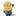## reading data from i2c adc PCF8591

Hi, I'm using a PCF8591 to read voltage from a sensor(0 to 5V) and then display it via my MBED on the pc using tera term. The voltage is reading correctly, but at random it displays a wrong value....as shown below.(data upto 6 decimal places is necessary)

the voltage is 4.375000 the voltage is 4.375000 the voltage is 4.375000 the voltage is 4.375000 the voltage is 4.375000 the voltage is 4.375000 the voltage is 4.375000 the voltage is 4.375000 the voltage is 4.394531 the voltage is 4.375000 the voltage is 4.375000 the voltage is 4.375000 the voltage is 4.375000 the voltage is 4.394531

The MBED code I have written is as below

```#include "mbed.h"
Serial pc(USBTX, USBRX);
I2C i2c(p28, p27);

int main()
{
char data,vdata;
float a,b;
data=0x02;/*selecting AIN2 for PCF 8591 */
i2c.start();
i2c.write(0x48,data,1); /* wrong address given beacause of some bug in i2c.*/
i2c.write(0x90,data,1);

while(1)
{
i2c.read(0x90,vdata,1);
wait(0.3);
b=vdata;
a=(b/256)*5;
pc.printf("\n the voltage is %f ",a);
}
}
```

How can i rectify the error. i.e. only one constant value needs to be read.

The address isnt given wrong, and neither is there a bug in i2c, different definitions are used. 0x48 is the 7-bit address, 0x90 is the 8-bit address that mbed uses.

Anyway considering it is an 8-bit ADC it is perfectly normal it can sometimes be one bit higher. There are many reasons why that can happen, you dont know exactly what you input voltage is, maybe it is exactly between the two voltages you read there, and there is always some noise. So if the difference is so small it isnt an error.

####Erik - wrote:

The address isnt given wrong, and neither is there a bug in i2c, different definitions are used. 0x48 is the 7-bit address, 0x90 is the 8-bit address that mbed uses.

the 0x48 can be replaced by any number. The thing is if i do not include that line, the PCF8591 will not read anything, instead it will by default send the data from AIN0 (analog input 0).

Hi..

sitting here with an application board, I wondered how stable my multimeter was in comparison. So, I put it across a 5v rail on the board.

I noticed it was dipping by 0.02v every 5 secs. That's odd I thought.. I thought my multimeter would be stable, and why so regular ?

Then I noticed the dips coincided with an LED turning itself on and off on the board I was testing the 5v rail on.

then I thought..

0.02v on a 5v rail,.. presuming my multimeter is ok, that LM7805 (that's creating the 5 volts) is only holding the rail to within 0.4%.

Is it some such ? also 0.02v is pretty small in the real world when applied.

####Erik - wrote:

The address isnt given wrong, and neither is there a bug in i2c, different definitions are used. 0x48 is the 7-bit address, 0x90 is the 8-bit address that mbed uses.

the 0x48 can be replaced by any number. The thing is if i do not include that line, the PCF8591 will not read anything, instead it will by default send the data from AIN0 (analog input 0).

The device will not respond to 0x48. The problem that it ignores the next command to select AIN2 when you dont have the dummy write to 0x48 may be caused by the unnecessary i2c.start(). Note that i2c block writes and reads generate their own start and stop conditions.

So instead of

```..
data=0x02;/*selecting AIN2 for PCF 8591 */
i2c.start();
i2c.write(0x48,data,1); /* wrong address given beacause of some bug in i2c.*/
i2c.write(0x90,data,1);
...
```

try

```..
data=0x02;/*selecting AIN2 for PCF 8591 */

i2c.write(0x90,data,1);
...
```

You may also want to consider removing the repeated start option for the write and read methods since it doesnt add much in this case. Edit: forget that final remark, need more sleep and less coffee..

He doesnt have the repeated start option, that would be the 4th argument, this is just the number of bytes to write. But I wouldnt be surprised if you are correct about the first line being required due to the extra start command that shouldnt be there.